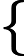Study of mathematics online.
Study math with us and make sure that "Mathematics is easy!"

# Decomposition of the vector in the basis

To decomposition, the vector b on the basis vectors a1, ..., an, you must find the coefficients of x1, ..., xn, for which a linear combination of vectors a1, ..., an is equal to vector b:

x1a1 + ... + xnan = b,

the coefficients x1, ..., xn are called the coordinates of the vector b in the basis a1, ..., an.

## Decomposition of the vector in the basis - example

Example 1. Decompose the vector b = {8; 1} by basis vectors p = {1; 2} and q = {3; 1}.

Solution: Form the vector equation:

xp + yq = b,

which can be written as a system of linear equations1x + 3y = 8 2x + 1y = 1

from the first equation express xx = 8 - 3y 2x + y = 1

Substitute x in the second equationx = 8 - 3y 2(8 - 3y) + y = 1x = 8 - 3y 16 - 6y + y = 1x = 8 - 3y 5y = 15x = 8 - 3y y = 3x = 8 - 3·3 y = 3x = -1 y = 3

Answer: b = -p + 3q.

Add the comment

 0